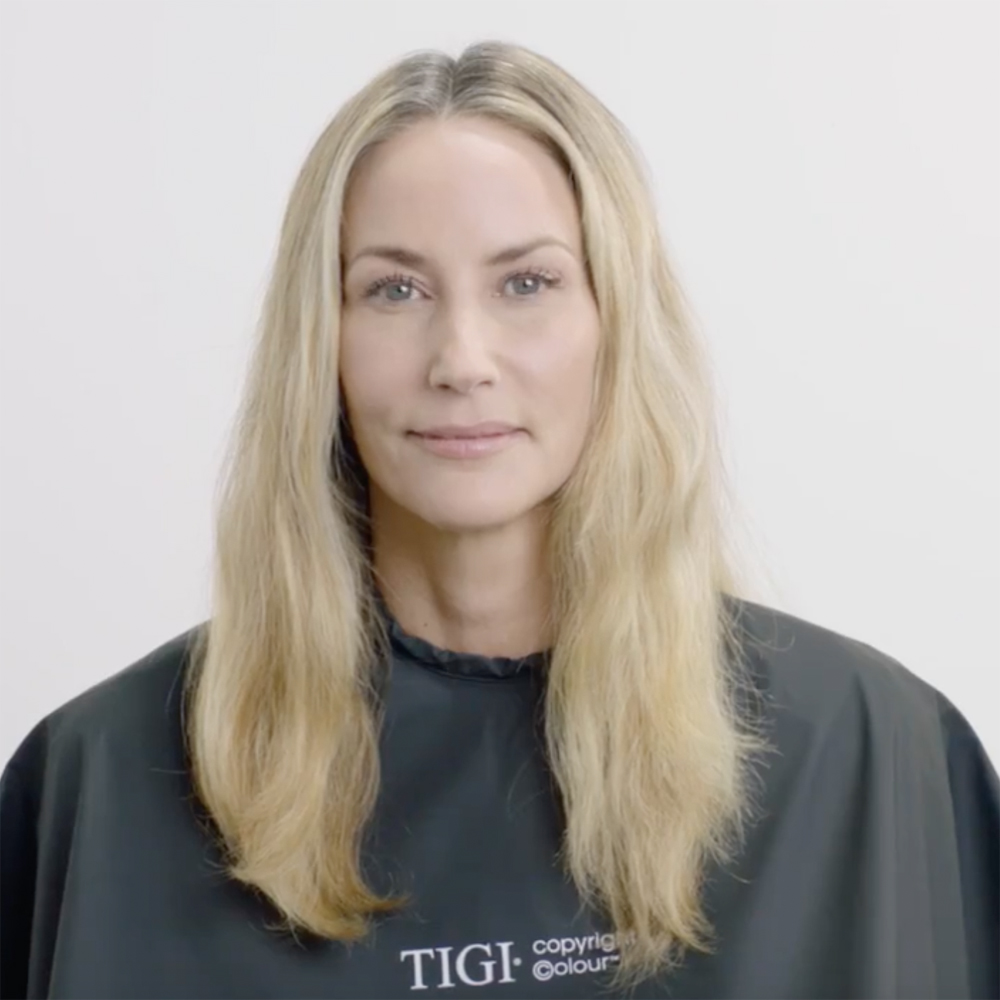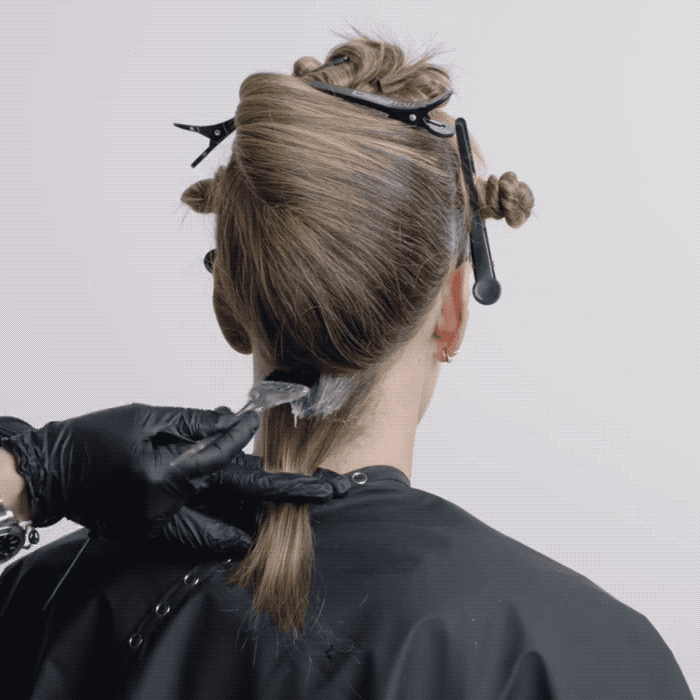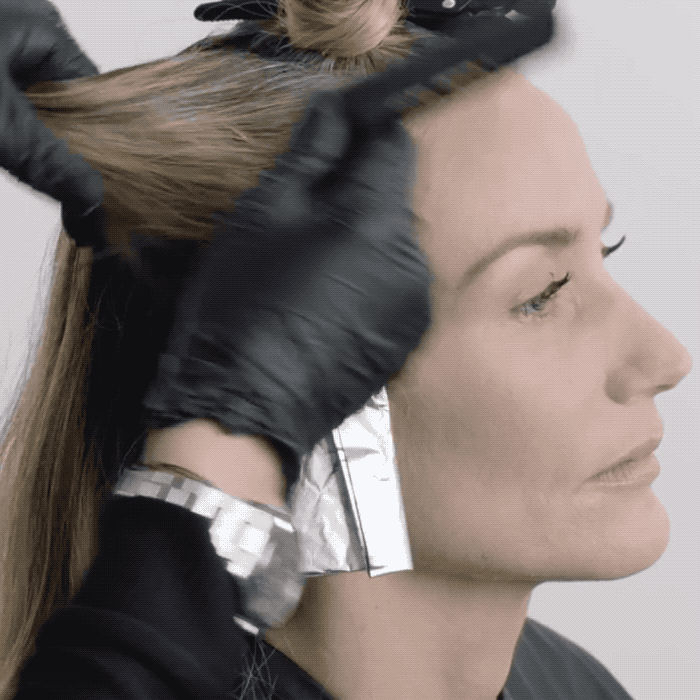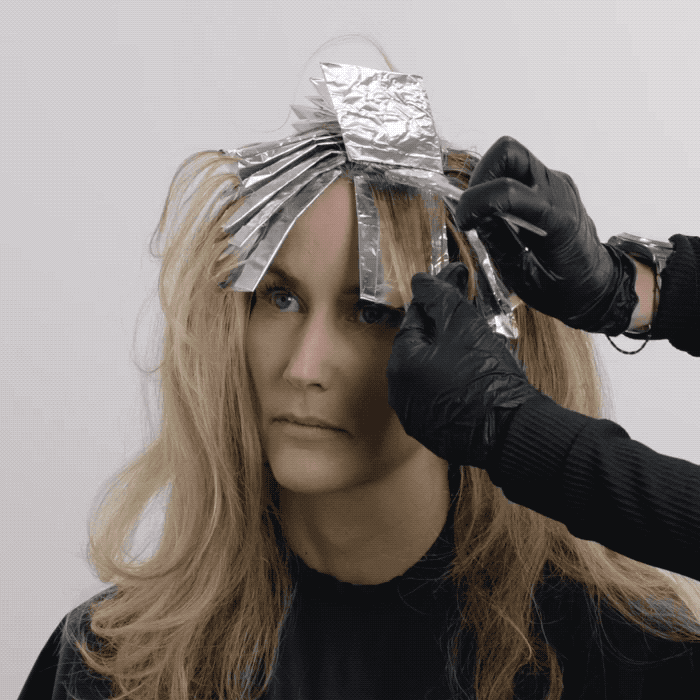Blonde Contouring + Gray Coverage
October 24, 2017

# Blonde Contouring + Gray Coverage

VIEW ALL PHOTOS VIEW SLIDESHOW
GET THE HOW-TO• Formula A

• Formula B

• Formula C# Blonde Contouring + Gray Coverage

No matter her age, your blondes want to stay blonde—so try this soft, multi-tonal effect that contours her face with feminine lines while also providing 100 percent gray coverage. The seamless highlights add dimension while softening the natural regrowth. Check out the technique from TIGI® Global Creative Technical Director Christel Lundqvist.

Colorist: Christel Lundqvist
Manufacturer: TIGI, @tigiprofessionals

• Formula A

• Formula B

• Formula C

## HOW-TO STEPS•• Formula A

• Formula B

• Formula C

1

Natural Level: 6

Percentage of non-pigmented hair: 50%

•• Formula A

• Formula B

• Formula C

2

Take a natural profile parting, then take a radial parting on each side of the head. Take a slight diagonal back parting on a high recession towards the crown, and repeat on the opposite side. This gives you five sections in total. Secure all sections with TIGI® Pro Sectioning Clips.

•• Formula A

• Formula B

• Formula C

3

Start the application at the nape area by taking horizontal partings. Apply Formula A to the new growth. Continue working in the same way until the back section is complete.

••• Formula A

• Formula B

• Formula C

4

Move to the side by taking a slight diagonal parting and a seamless weave to create a natural highlighting effect. Apply Formula B to the weave. Continue working up the side of the head in the same manner until the section is complete, then repeat on the opposite side.

•• Formula A

• Formula B

• Formula C

5

Apply Formula A to the new growth on either side.

•• Formula A

• Formula B

• Formula C

6

On the final, top section, take a diagonal parting and a seamless weave with Formula B. Repeat the same technique on the opposite side

•• Formula A

• Formula B

• Formula C

7

Apply Formula A to the new growth.

•• Formula A

• Formula B

• Formula C

8

Process for 30 minutes, then rinse and remove. Tone the prelightened areas with Formula C. Process and shampoo, then style as desired.

•• Formula A

• Formula B